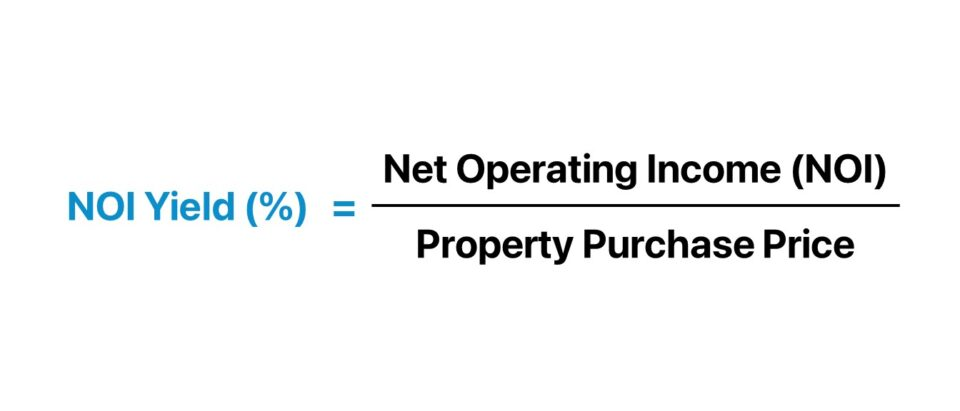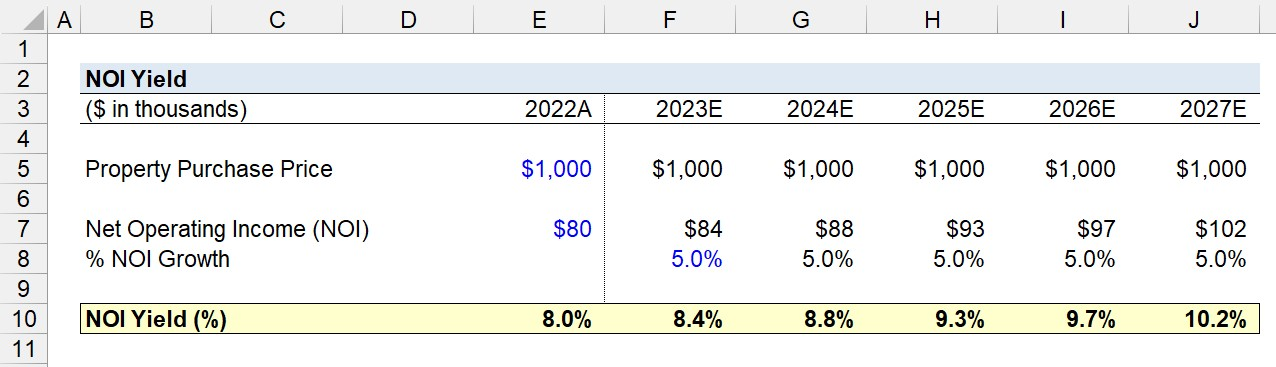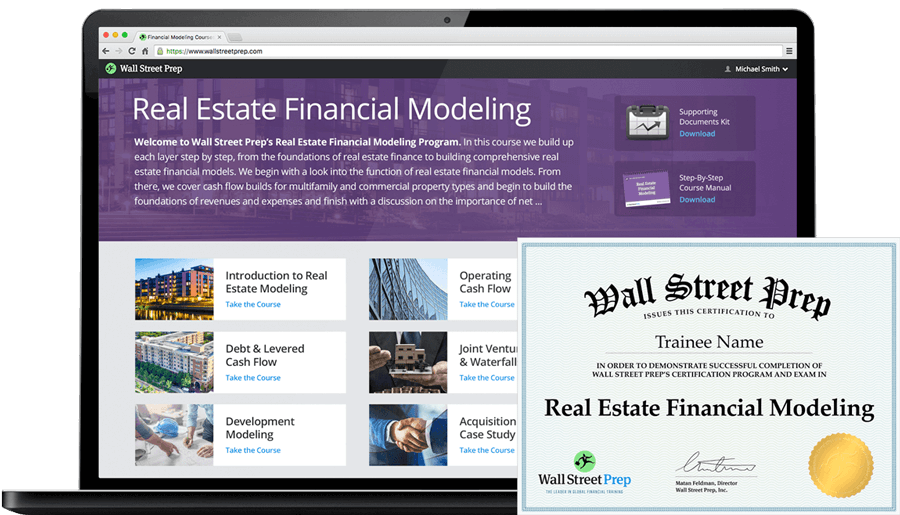Welcome to Wall Street Prep! Use code at checkout for 15% off.# NOI Yield

Guide to Understanding NOI Yield (%)## How to Calculate NOI Yield?

The NOI yield measures the annual profitability of a property investment relative to its initial purchase price.

The net operating income (NOI) of a property is the effective gross income (EGI) of a property, net of any direct operating expenses.

Effective Gross Income (EGI) = Potential Gross Income (PGI) Vacancy and Credit Losses
Net Operating Income (NOI) = Effective Gross Income (EGI) Direct Operating Expenses

Conversely, the formula to compute the net operating income (NOI) can be expressed as the following.

Net Operating Income (NOI) = (Rental Income + Ancillary Income) Direct Operating Expenses

The NOI yield represents the annual rate of return received by a real estate investor based on the income generated by the property and the purchase price paid to acquire the property.

Unlike the cap rate, which is usually computed as the NOI divided by the market value of the property, the NOI yield is the annual NOI divided by the total purchase price.

The purchase price includes not only the cost of acquiring the property, but also any other transaction-related expenses like consulting fees, appraisal fees, etc.## NOI Yield Formula

The formula to calculate the NOI yield is as follows.

NOI Yield (%) = Net Operating Income (NOI) ÷ Property Purchase Price

Where:

• Net Operating Income (NOI) → Total Income (Rental Income + Ancillary Income) Less Direct Operating Expenses
• Property Purchase Price → Original Cost of Purchasing Property

Since the numerator is NOI, an unlevered metric – rather than cash flow after debt service (CFADS) – the output reflects the “unlevered” yield on property investments.

## What is the Difference Between the NOI Yield vs. Cap Rate?

The NOI yield measures the annual return received by a real estate investor based on the price paid to purchase a property.

The priority of the NOI yield is to understand the return attributable to income, as opposed to capital appreciation.

The cap rate, or “capitalization rate”, is calculated by dividing the net operating income (NOI) of a property by its current market value, whereas the NOI yield divides NOI by the total purchase price.

Cap Rate (%) = Net Operating Income (NOI) ÷ Market Value of Property

Hence, the cap rate fluctuates based on changes in the property’s market value. However, the NOI yield utilizes the total cost as of the date of original purchase, which is a constant figure.

The more that the market value of the property changes over time, the more that the cap rate and NOI yield of the property will drift apart – all else being equal.

## NOI Yield Calculator

We’ll now move to a modeling exercise, which you can access by filling out the form below.#### Excel TemplateSubmitting...

## NOI Yield Calculation Example

Suppose a real estate investment firm acquired a commercial property for \$1 million near the end of 2021.

By the start of 2022, the minor repair work and renovations are complete, and the property has reached a state of stabilization, in which the property is fully functional and generates income.

In 2022, the property generated \$80k in net operating income (NOI), and for the next five years, the property’s NOI is projected to increase by 5.0% per year.

• Property Purchase Price = \$1 million
• Net Operating Income (NOI), 2022A = \$80k
• NOI Growth (%) = 5.0% Per Year (5-Year Projection Period)

Initially, the NOI yield in 2022 starts at 8.0%. But over the five-year forecast period – where the NOI of the property grows by 5.0% per year – the implied NOI yield reaches 10.2% by the end of 2027.

• NOI Yield (%), 2022A = \$80k ÷ \$1 million = 8.0%
• NOI Yield (%), 2023E = \$84k ÷ \$1 million = 8.4%
• NOI Yield (%), 2024E = \$88k ÷ \$1 million = 8.8%
• NOI Yield (%), 2025E = \$93k ÷ \$1 million = 9.3%
• NOI Yield (%), 2026E = \$97k ÷ \$1 million = 9.7%
• NOI Yield (%), 2027E = \$102k ÷ \$1 million = 10.2%

In conclusion, the expansion in the NOI yield from 8.0% in 2022 to 10.2% in 2027 is attributable to the growth in the property’s net operating income (NOI).20+ Hours of Online Video Training

### Master Real Estate Financial Modeling

This program breaks down everything you need to build and interpret real estate finance models. Used at the world's leading real estate private equity firms and academic institutions.

Inline Feedbacks